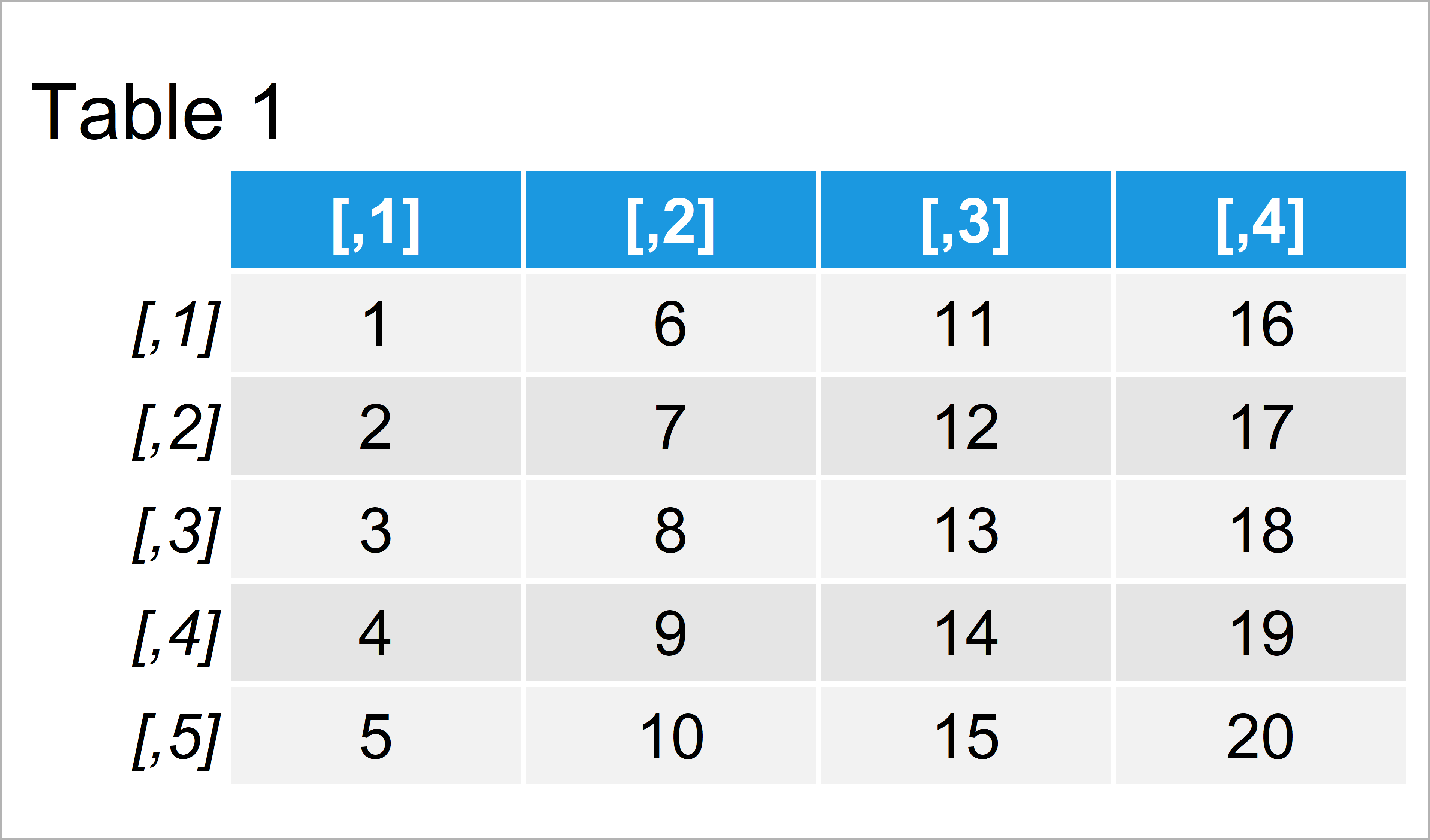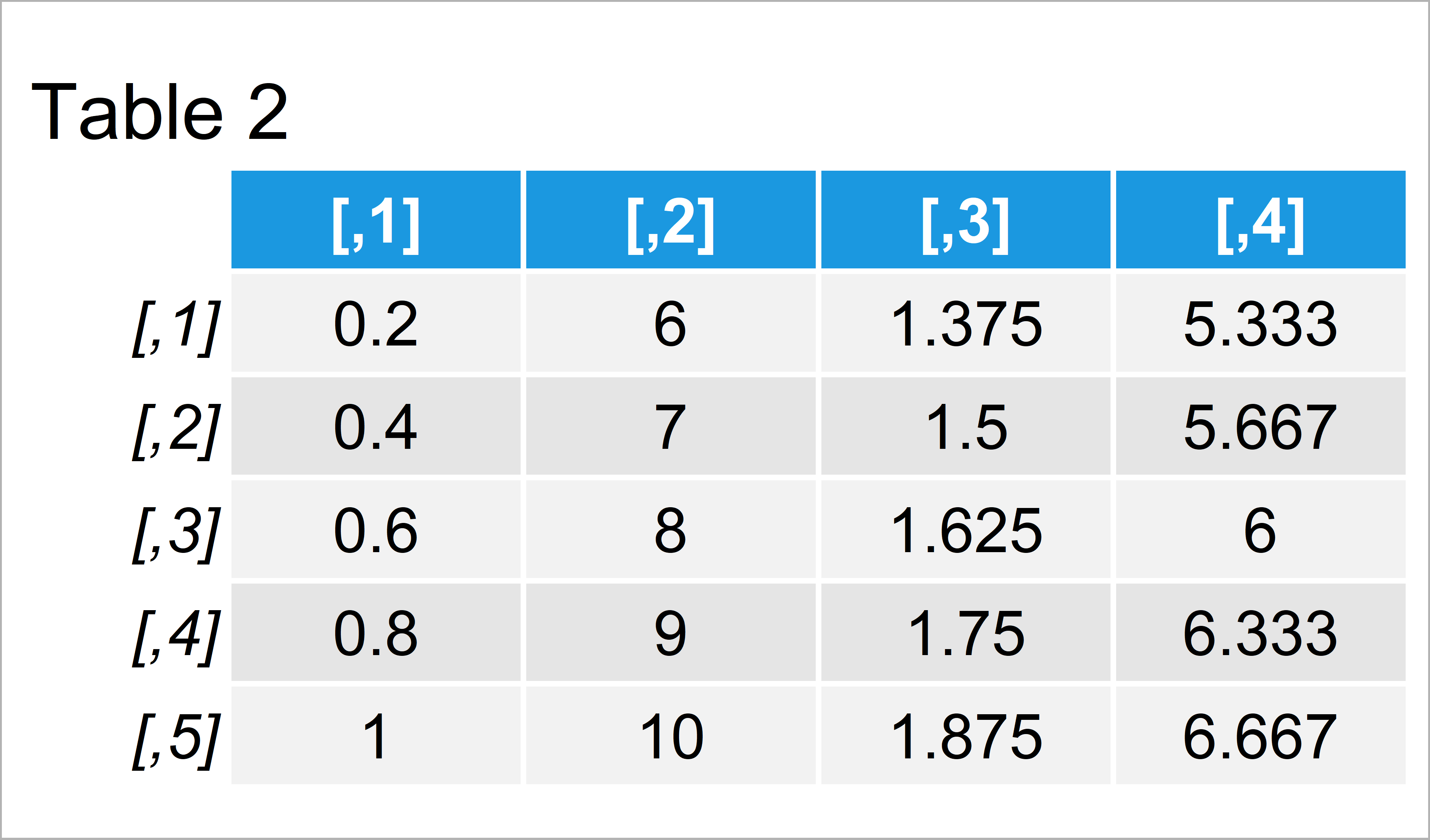# Divide Each Row of Matrix & Data Frame by Vector Elements in R (2 Examples)

This tutorial explains how to divide the rows of a data matrix by the elements of a vector in R.

The tutorial will contain the following information:

Let’s start right away.

## Example 1: Divide Each Row of Matrix by Elements of Vector

This example illustrates how to divide each row of a matrix by the elements of a vector object in the R programming language.

First, we have to create an exemplifying matrix in R:

```mat <- matrix(1:20, ncol = 4)                  # Create example matrix
mat                                            # Print example matrix```The output of the previous R programming code is shown in Table 1: We have created a numeric matrix with five rows and four columns.

Next, we have to create a vector object:

```vec <- c(5, 1, 8, 3)                           # Create example vector
vec                                            # Print example vector
#  5 1 8 3```

Our vector contains four numeric elements, i.e. the same length as the number of columns of our matrix.

Now, we can use the t() function (t stands for transpose) and the / operator to divide each row of our matrix by the elements of our vector:

```mat_div <- t(t(mat) / vec)                     # Divide rows of matrix by vector
mat_div                                        # Print divided matrix```By executing the previous syntax we have created Table 2, i.e. a new matrix object containing of the results of the divisions.

## Example 2: Divide Each Row of Data Frame by Elements of Vector

In Example 2, I’ll explain how to perform a mathematical division of each row of a data frame by the elements of a vector.

For this, we can convert our matrix that we have created in Example 1 to the data.frame class by using the as.data.frame function.

```data <- as.data.frame(mat)                     # Create example data frame
data                                           # Print example data frame```In Table 3 it is shown that we have created a data frame containing the same values as our example matrix that we have used in Example 1.

Now, we can use basically the same code as in Example 1 to divide each row of our data frame by a vector. The only difference is that we are using the as.data.frame function once again to return the results in a data frame object as well.

Have a look at the following R code and its output:

```data_div <- as.data.frame(t(t(data) / vec))    # Divide rows of data frame by vector
data_div                                       # Print divided data frame```The output of the previous R programming syntax is shown in Table 4: A data frame containing the results of our matrix/vector division.

## Video & Further Resources

Do you need more explanations on the content of this tutorial? Then you might want to watch the following video of my YouTube channel. I explain the R programming codes of this tutorial in the video:

Please accept YouTube cookies to play this video. By accepting you will be accessing content from YouTube, a service provided by an external third party.If you accept this notice, your choice will be saved and the page will refresh.

Furthermore, you might want to have a look at some of the related tutorials of my website. I have released several articles about matrix calculations already.

Summary: At this point you should know how to use the t() function to divide rows by a vector in R. If you have further questions, let me know in the comments section.

Subscribe to the Statistics Globe Newsletter